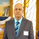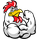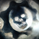5670 vizualizações
*** April 20, 2015 - NEW UPDATE ***

April 10, 2015 - Updated version of Value Chart - candles draw correctly now.
Requires cover layer to be set as same color as your background. (white in default)

I hope you will enjoy it. :)
```//Created by TheMightyChicken
//Based on LazyBear's Value Chart
study("Value Chart [TheMightyChicken]", shorttitle="VC")
// Inputs
length = input(5, title="Number of bars")
extTop = input(8, title="Extreme level top")
extBot = input(-8, title="Extreme level bottom")
// Value Chart Calculations
prev(s,i) =>
y=abs(round(i))
s[y]
varp = round(length/5)
h_f = length > 7
vara=h_f ? highest(high,varp)-lowest(low,varp) : 0
varr1 = h_f ? iff(vara==0 and varp==1,abs(close-prev(close,-varp)),vara) : 0
varb=h_f ? prev(highest(high,varp),-varp+1)-prev(lowest(low,varp),-varp) : 0
varr2 = h_f ? iff(varb==0 and varp==1,abs( prev(close,-varp)-prev(close,-varp*2) ),varb) : 0
varc=h_f ? prev(highest(high,varp),-varp*2)-prev(lowest(low,varp),-varp*2) : 0
varr3 = h_f ? iff(varc == 0 and varp==1,abs(prev(close,-varp*2)-prev(close,-varp*3)),varc) : 0
vard = h_f ? prev(highest(high,varp),-varp*3)-prev(lowest(low,varp),-varp*3) : 0
varr4 = h_f ? iff(vard == 0 and varp==1,abs(prev(close,-varp*3)-prev(close,-varp*4)),vard)  : 0
vare = h_f ? prev(highest(high,varp),-varp*4)-prev(lowest(low,varp),-varp*4) : 0
varr5 = h_f ? iff(vare == 0 and varp==1,abs(prev(close,-varp*4)-prev(close,-varp*5)),vare) : 0
cdelta = abs(close - prev(close,-1))
var0 = (not h_f) ? iff((cdelta > (high-low)) or (high==low),cdelta,(high-low)) : 0
lrange=h_f ? ((varr1+varr2+varr3+varr4+varr5)/5)*.2 : sma(var0,5)*.2
mba = sma( (high+low)/2,length)
vOpen = (open-mba)/lrange
vClose = (close-mba)/lrange
vHigh = (high-mba)/lrange
vLow = (low-mba)/lrange
// Body Calculations
bodyHigh = vOpen>vClose ? vOpen : vClose
bodyLow = vOpen<vClose ? vOpen : vClose
body0 = (vOpen>0 and vClose>0) or (vOpen>0 and vClose<0) ? bodyHigh : (vOpen<0 and vClose<0) or (vOpen<0 and vClose>0) ? bodyLow : 0
body1 = vOpen<0 and vClose>0 ? bodyHigh : vOpen>0 and vClose<0 ? bodyLow : 0
bodyCover = vOpen>0 and vClose>0 ? bodyLow : vOpen<0 and vClose<0 ? bodyHigh : 0
// Wick Calculations
wick0 = vHigh>0 ? vHigh : vLow
wick1 = vHigh>0 and vLow<0 ? vLow : vHigh
wickCover = vHigh>0 and vLow>0 ? vLow : vHigh<0 and vLow<0 ? vHigh : 0
// Plots
vcolor = vClose>extTop ? lime : vClose<extBot ? red : silver
top_l = plot(extTop, title="Extreme Top Line", color=silver)
bot_l = plot(extBot, title="Extreme Bottom Line", color=silver)
fill(top_l, bot_l, black, transp=95)
plot(body0, linewidth=3, color=vcolor, style=histogram)
plot(body1, linewidth=3, color=vcolor, style=histogram)
plot(bodyCover, linewidth=3, color=white, style=histogram)
plot(wick0, linewidth=1, color=vcolor, style=histogram)
plot(wick1, linewidth=1, color=vcolor, style=histogram)
plot(wickCover, linewidth=1, color=white, style=histogram)
```

## ComentáriosWhat is S and I here? prev(s,i) =>
y=abs(round(i))
s
Thanks.
RespostaLast version of value chart. You can set your own length from now. :)
RespostaHollow candles version. Play with fix value to adjust pixels. ;)
source code: http://pastebin.com/hFkY3NB3
Resposta*** 20.4.2015 - NEW UPDATE ***
RespostaGood Job.

Just a suggestion, Force the background color to white. Therefore, it makes it easier for those who don't understand. If you chose to make it optional so, people still dont have to sacrifice their preferred layout bgcolor.
RespostaGood Job.

Just a suggestion, Force the background color to white. Therefore, it makes it easier for those who don't understand. If you chose to make it optional so, people still dont have to sacrifice their preferred layout bgcolor.
RespostaI will appreciate if you can some information on how to interpret this indicator! thanks!
Respostadawarravi
There are 100s of videos on youtube, Search for Mark Helweg Value Charts. you can watch the entire series of Marketing videos.
RespostaVery nice...I really like the way you've used histo...great job!

Looking forward to your other scripts.
Resposta